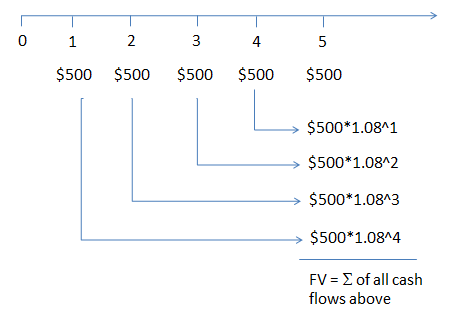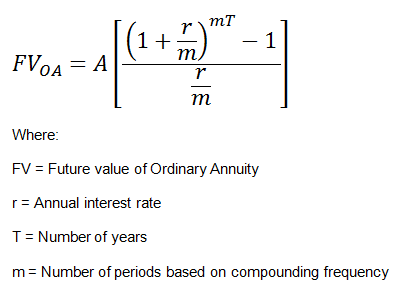# Future Value and Present Value of Ordinary Annuity

An annuity refers to a series of equal cash flows that occur periodically such as monthly, quarterly or annually. For example, an investment that gives you fixed monthly payments for a specified period. There are two types of annuities, namely, ordinary annuities and annuities due. .

In an ordinary annuity, the first cash flow occurs at the end of the first period, and in an annuity due, the first cash flow occurs at the beginning (at time 0).

The present value and future values of these annuities can be calculated using a simple formula or using the calculator.

### Future Value of an Ordinary Annuity

Let’s say we have an ordinary annuity that pays \$500 every year for the next 5 years. The expected rate of return is 8%. The future value of this annuity can be represented as follows:This can be calculated using the following formula:While you can use the above formula to calculate the future value of annuity, you can simply calculate the future value using the BAII Plus calculator. Note that in our example, m = 1, since the compounding frequency is 1.